# Mathematica提取边缘座标2018年3月27日06:08:10

1 965字阅读3分13秒

``````data = Flatten[Table[{x, x + 5*Sin[x] + RandomReal[2*j]}, {x, 0, 10, .1}, {j, 1, 10}], 1];
ListPlot[data]``````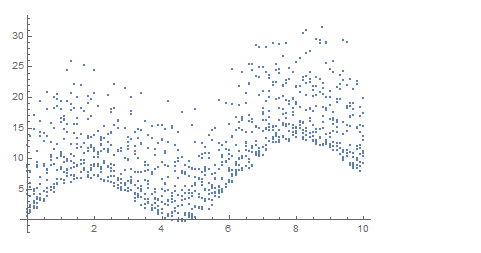``Show[ConvexHullMesh[data], Graphics[Point[data]]]``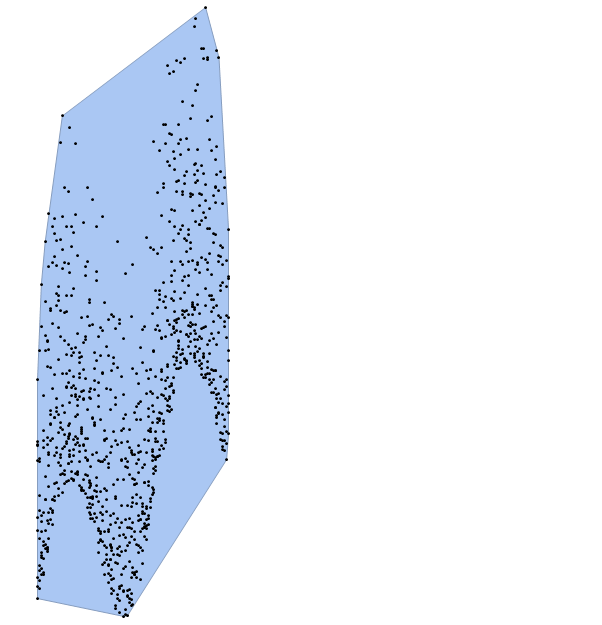``````zb = MeshCoordinates[ConvexHullMesh[data]];
Length[zb]
>> 30``````

``Show[ConvexHullMesh[data], Graphics[{Red, PointSize[Large], Point[zb]}]]``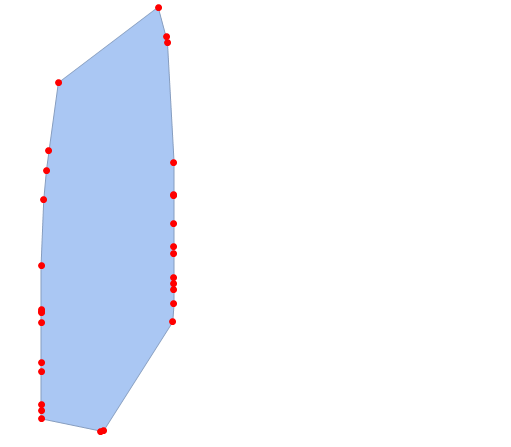``````SortBy[zb, #[] &][[;; 2]]
>> {{4.5, -0.320249}, {4.7, -0.298881}}``````

``````zb2 = GatherBy[zb, #[] > 4.5 &]
Show[ConvexHullMesh[data],
Graphics[{Red, PointSize[Large], Point[zb2[]]}],
Graphics[{Green, PointSize[Large], Point[zb2[]]}]]``````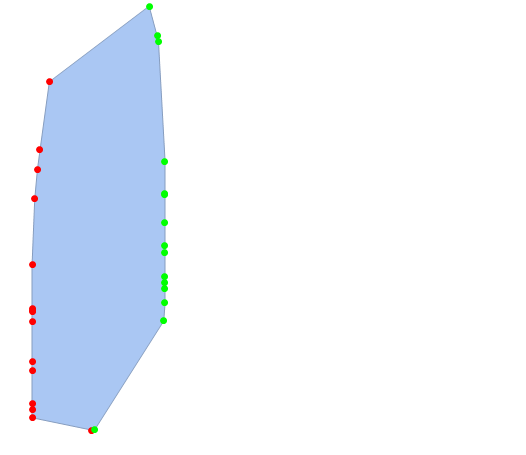``````maxzb = MaximalBy[#, #[] &] & /@ zb2
>> {{{1.3, 25.8798}}, {{8.8, 31.5633}}}
Show[ConvexHullMesh[data],
Graphics[{Red, PointSize[.2], Point[maxzb[]]}],
Graphics[{Green, PointSize[.2], Point[maxzb[]]}]]``````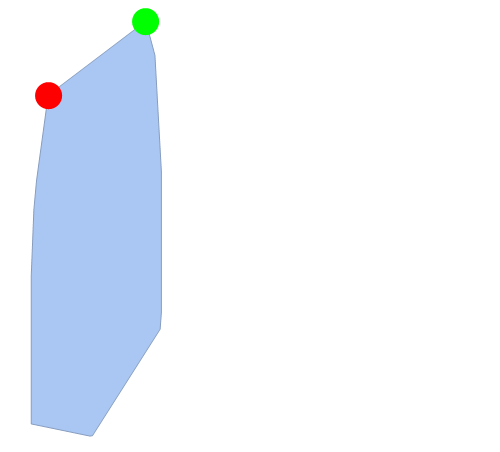• 微信公众号
• 关注微信公众号
•• QQ群
• 我们的QQ群号
•• 本文由 发表于 2018年3月27日06:08:10
• 转载请务必保留本文链接：https://mathpretty.com/9089.html